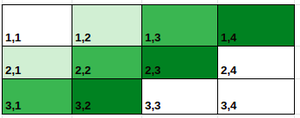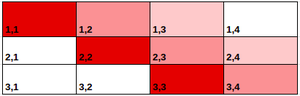# Minimum number of hint required to get the hidden cell in 2D grid

• Last Updated : 13 Jan, 2022

Given a 2D array of size M * N. The task is to find the minimum number of hint required to pick the correct position of a hidden cell on the grid, where in each hint the Manhattan distance of hidden cell from any cell of one’s choice will be informed.

Note: Manhattan distance between two cells is the sum of absolute differences between rows and columns of the cells.

Examples:

Input: M = 1, N = 3
Output: 1
Explanation: For any hidden cell , if X-path distance from (1,1) is known, then hidden cell is (1, 1+X).

Input: M = 1, N = 1
Output: 0
Explanation: Only one possible cell.

Approach: There are three possible cases to find the position of cell on the grid:

Case-1: When grid is of row form i.e.  dimension =  (1 x N)

For the above table, a minimum of one hint is required. In the hint the distance from cells(1, 1) can be obtained and if X is the distance of the hidden cell from (1, 1) then (1, 1+X ) will be the hidden cell.

Case-2: When grid is of column form i.e.  dimension =  (N x 1)

For the above table, a minimum of one hint is required. In the hint the distance from (1, 1) can be obtained and if X is the distance of the hidden cell from (1, 1) then (1+X, 1) will be the hidden cell.

Case-3: When the grid is of rectangle form i.e.  dimension =  (M x N)
For this type of tables, a minimum of two hints are required. The hints required are shown below:

1. One to get the path distance from cell (1, 1) and if X is the path distance of the hidden cell from (1, 1) then any cell of form (1+X1, 1+X2) will be the hidden cell where X1 + X2 = X.
2. Other one to get the path distance from cell (1, N) and if Y is the path distance of the hidden cell from (1, N) then any cell of form (1+Y1, N-Y2) will be the hidden cell where Y1 + Y2 = Y.

For any combination of X and Y there is only one cell which satisfies both the distance. Using the two above equations the hidden cell can be found easily. So, in this case, a minimum of two pieces of help will be needed.

Follow the image below for better understanding of the conditions and intersection of the cells with any X and Y values. Any value of X and Y will have only one cell as the intersection point.equi distant cells from 1,1equiDistant cell from (1,N) i.e 1,4

Below is the implementation of the above approach:

## C++

 `// C++ code to implement above approach``#include ``using` `namespace` `std;` `// Function to find minimum help required``// for finding the hidden cell``int` `minHints(``int` `M, ``int` `N)``{``    ``int` `res;` `    ``// Grid having one cell``    ``if` `(M == 1 && N == 1)``        ``res = 0;` `    ``// Row form or column form grid``    ``else` `if` `(M == 1 || N == 1)``        ``res = 1;` `    ``// Rectangle form grid``    ``else``        ``res = 2;` `    ``return` `res;``}` `int` `main()``{``    ``// Declaring the dimension of the grid``    ``int` `M = 1, N = 3;` `    ``cout << minHints(M, N);``    ``return` `0;``}`

## Java

 `// Java code to implement above approach``import` `java.util.*;``public` `class` `GFG``{` `  ``// Function to find minimum help required``  ``// for finding the hidden cell``  ``static` `int` `minHints(``int` `M, ``int` `N)``  ``{``    ``int` `res;` `    ``// Grid having one cell``    ``if` `(M == ``1` `&& N == ``1``)``      ``res = ``0``;` `    ``// Row form or column form grid``    ``else` `if` `(M == ``1` `|| N == ``1``)``      ``res = ``1``;` `    ``// Rectangle form grid``    ``else``      ``res = ``2``;` `    ``return` `res;``  ``}` `  ``public` `static` `void` `main(String args[])``  ``{``    ` `    ``// Declaring the dimension of the grid``    ``int` `M = ``1``, N = ``3``;` `    ``System.out.print(minHints(M, N));``  ``}``}` `// This code is contributed by Samim Hossain Mondal.`

## Python3

 `# python3 code to implement above approach` `# Function to find minimum help required``# for finding the hidden cell``def` `minHints(M, N):``    ``res ``=` `0` `    ``# Grid having one cell``    ``if` `(M ``=``=` `1` `and` `N ``=``=` `1``):``        ``res ``=` `0` `    ``# Row form or column form grid``    ``elif` `(M ``=``=` `1` `or` `N ``=``=` `1``):``        ``res ``=` `1` `    ``# Rectangle form grid``    ``else``:``        ``res ``=` `2` `    ``return` `res`  `if` `__name__ ``=``=` `"__main__"``:` `    ``# Declaring the dimension of the grid``    ``M ``=` `1``    ``N ``=` `3` `    ``print``(minHints(M, N))` `    ``# This code is contributed by rakeshsahni`

## C#

 `// C# program for the above approach``using` `System;``class` `GFG``{` `  ``// Function to find minimum help required``  ``// for finding the hidden cell``  ``static` `int` `minHints(``int` `M, ``int` `N)``  ``{``    ``int` `res;` `    ``// Grid having one cell``    ``if` `(M == 1 && N == 1)``      ``res = 0;` `    ``// Row form or column form grid``    ``else` `if` `(M == 1 || N == 1)``      ``res = 1;` `    ``// Rectangle form grid``    ``else``      ``res = 2;` `    ``return` `res;``  ``}` `  ``public` `static` `void` `Main()``  ``{` `    ``// Declaring the dimension of the grid``    ``int` `M = 1, N = 3;` `    ``Console.Write(minHints(M, N));``  ``}``}` `// This code is contributed by Samim Hossain Mondal.`

## Javascript

 ``

Output
`1`

Time Complexity: O(1)
Auxiliary Space: O(1)

My Personal Notes arrow_drop_up